Madagascar Programming Reference ManualNext: Products of operators Up: Introduction Previous: Introduction

## Definition of operators

Mathematically speaking an operator is a function of a function, i.e. a rule (or mapping) according to which a functionis transformed into another function. We use the notationor simply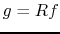, wheredenotes the operator. Examples of operators are the derivative, the integral, convolution (with a specific function), multiplication by a scalar and others. Note that in general the domains ofandare not necessarily the same. For example, in the case of the derivative, the domain ofis the subset of the domain of, in whichis smooth. In particular if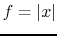,, then the domain ofis.

An important class of operators are the linear operators. An operatoris linear if for any two functions,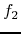and any two scalars,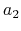,. The derivative, integral, convolution and multiplication by scalar are all linear operators.

In the discrete world, operators act on vectors and linear operators are in fact matrices, with which the vectors are multiplied. (Multiplication by a matrix is a linear operation, since). In fact many of the calculations performed routinely in science and engineering are essentially matrix multiplications in disguise. For example assume a vectorwith length(superscriptdenotes transpose). Padding this vector withzeros, produces another vectorwith

 whereis the zero vector of length. One can readily verify that zero padding is a linear operation with operator matrix, whereis theidentity matrix andis thezero matrix, sinceNote that as in the case of functions, the domains ofandare different: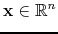(or more generally), while(or).

Similarly, one can define convolution ofwithas the multiplication ofwithand many other operations as matrix multiplications. Other operators are the identity operator is the identity matrixand is implemented by [sec:copy]copy.c and [sec:ccopy]ccopy.c and the null operator (or zero matrix), which is implemented by [sec:adjnull]adjnull.c. For the rest of this introduction, the boldface notation will imply specifically discrete operators, while the normal fonts will imply operators on either continuous or discrete mathematical entities.Madagascar Programming Reference ManualNext: Products of operators Up: Introduction Previous: Introduction

2011-07-02